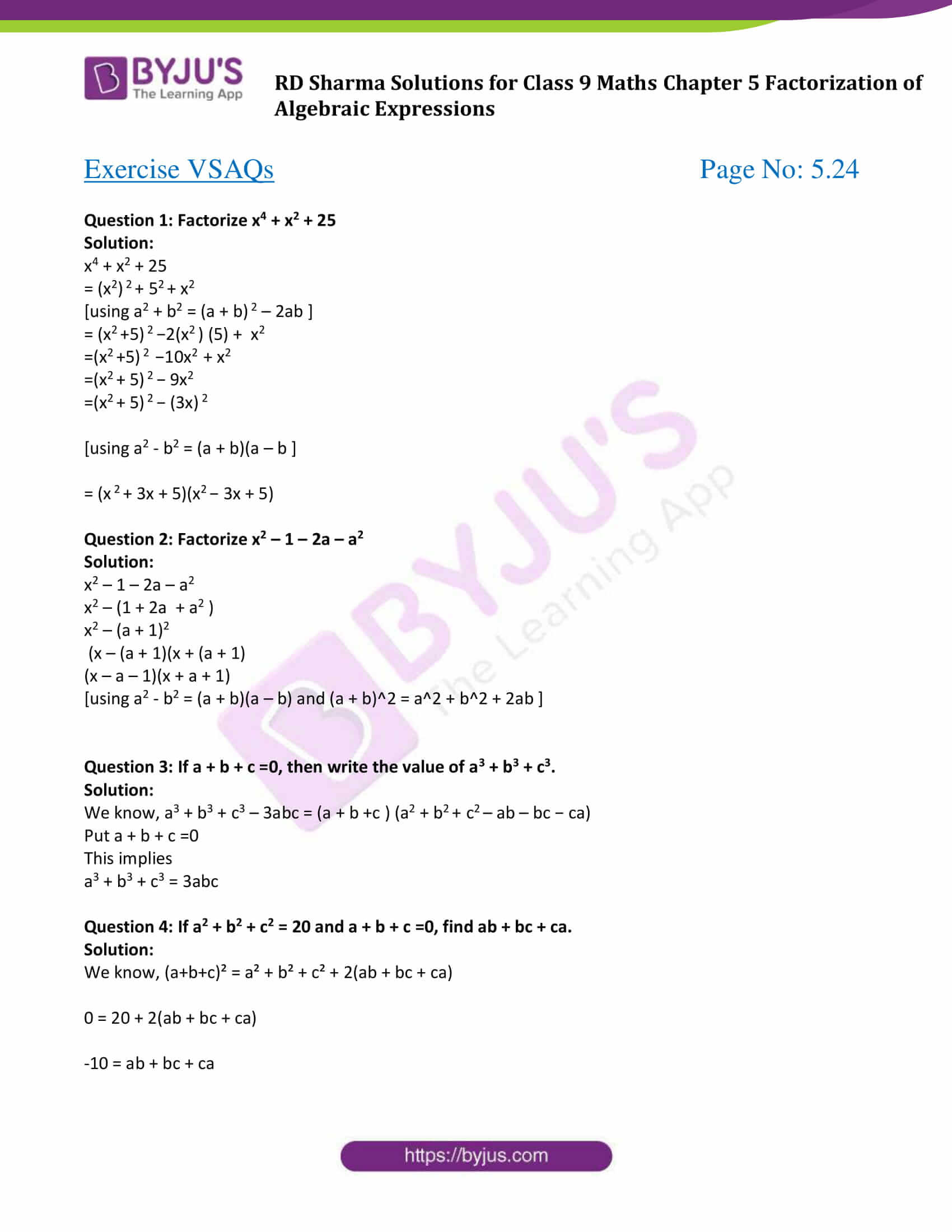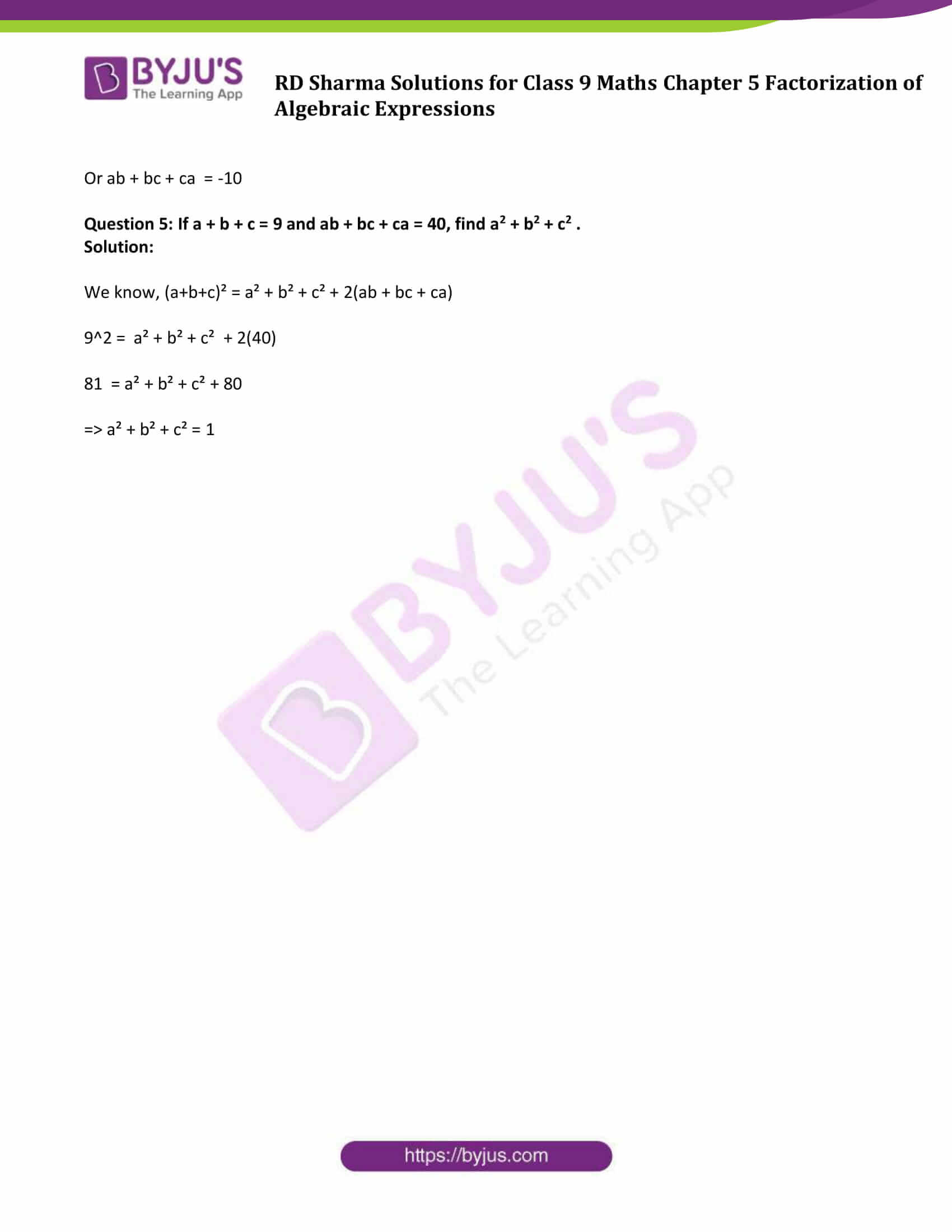# RD Sharma Solutions for Class 9 Maths Chapter 5 Factorization of Algebraic Expressions Exercise VSAQs

Factorization in Mathematics is breaking down of a Mathematical object into a product of other factors, which when multiplied together it gives the original. Here students will be able to learn how to factorize algebraic equation through solved RD Sharma Solutions class 9. The contents are written in a simpler manner and can be easily grasped. The RD Sharma solutions can be download from the link given below.

## Download PDF of RD Sharma Solutions for Class 9 Maths Chapter 5 Factorization of Algebraic Expressions Exercise VSAQs### Access Answers to Maths RD Sharma Solutions for Class 9 Chapter 5 Factorization of Algebraic Expressions Exercise VSAQs Page number 5.24

#### Exercise VSAQs Page No: 5.24

Question 1: Factorize x4 + x2 + 25

Solution:

x4 + x2 + 25

= (x2) 2 + 52 + x2

[using a2 + b2 = (a + b) 2 – 2ab ]

= (x2 +5) 2 −2(x2 ) (5) + x2

= (x2 +5) 2 −10x2 + x2

= (x2 + 5) 2 − 9x2

= (x2 + 5) 2 − (3x) 2

[using a2 – b2 = (a + b)(a – b ]

= (x 2 + 3x + 5)(x2 − 3x + 5)

Question 2: Factorize x2 – 1 – 2a – a2

Solution:

x2 – 1 – 2a – a2

x2 – (1 + 2a + a2 )

x2 – (a + 1)2

(x – (a + 1)(x + (a + 1)

(x – a – 1)(x + a + 1)

[using a2 – b2 = (a + b)(a – b) and (a + b)^2 = a^2 + b^2 + 2ab ]

Question 3: If a + b + c =0, then write the value of a3 + b3 + c3.

Solution:

We know, a3 + b3 + c3 – 3abc = (a + b +c ) (a2 + b2 + c2 – ab – bc − ca)

Put a + b + c =0

This implies

a3 + b3 + c3 = 3abc

Question 4: If a2 + b2 + c2 = 20 and a + b + c =0, find ab + bc + ca.

Solution:

We know, (a+b+c)² = a² + b² + c² + 2(ab + bc + ca)

0 = 20 + 2(ab + bc + ca)

-10 = ab + bc + ca

Or ab + bc + ca = -10

Question 5: If a + b + c = 9 and ab + bc + ca = 40, find a2 + b2 + c2 .

Solution:

We know, (a+b+c)² = a² + b² + c² + 2(ab + bc + ca)

92 = a² + b² + c² + 2(40)

81 = a² + b² + c² + 80

⇒ a² + b² + c² = 1

## RD Sharma Solutions for Class 9 Maths Chapter 5 Factorization of Algebraic Expressions Exercise VSAQs

RD Sharma Solutions Class 9 Maths Chapter 5 Factorization of Algebraic Expressions Exercise VSAQs is based on the following topics:

• Factorisation by grouping the terms.
• Factorisation by making a perfect square.
• Factorisation by the difference of two squares.
• Factorisation of algebraic expressions expressible as the sum or difference of two cubes.